Altair® Panopticon

# Percentile

The selected percentile.

Percentile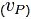is the value of the P-th percentile of an ascending ordered data set containing N elements with values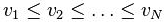.

There are two steps to compute for Percentile.

## Computing the Percentile

1. Calculate the rank: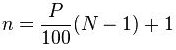The rank is then split into its integer component k and decimal component d, such that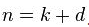.

1. Use the formula below to calculate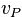as: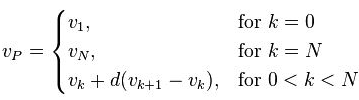Sample 1:

Consider the ordered list of values 15, 20, 35, 40, 50. What is the 40th percentile of this list?

## Computing the Percentile

1. Calculate the rank of the 40th percentile as follows: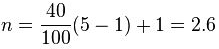Thus, n=2.6, which gives us k=2 and d=0.6.

1. Calculate the value of the 40th percentile.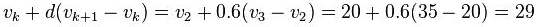Thus, the value of the 40th percentile of the ordered list 15, 20, 35, 40, 50 is 29.

Sample 2:

Consider the ordered list 1,2,3,4. What is the 75th percentile of this list?

## Computing the Percentile

1. Calculate the rank of the 75th percentile as follows.

N = 75/100(4-1) + 1 = 3.25

Thus, n=3.25, which gives us k=3 and d=0.25.

1. Calculate the value of the 75th percentile.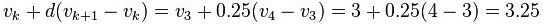Thus, the value of the 75th percentile of the ordered list 1,2,3,4 is 3.25.

Sample 3:

Given the following sample fields:

 Number Arbitrary Negative Values Positive Values One Binary Currency Decimal 1 3 -1 1 1 0 \$1.00 1.01 2 2 -2 2 1 \$10.00 2.02 3 3 -3 3 0 \$100.00 3.03 4 0 -4 4 1 \$1,000.00 4.04 5 -1 -5 5 0 \$10,000.00 5.05 6 -2 -6 6 1 -\$1.00 6.06 7 -3 -7 7 0 -\$10.00 7.07 8 0 -8 8 -\$100.00 8.08 9 0 -9 9 -\$1,000.00 9.09 10 0 -10 0 \$0.00 0.00

Sample fields

The 50th Percentile value for each field in the table:

 Number Arbitrary Negative Values Positive Values One Binary Currency Decimal 5.50 0.00 -5.50 4.50 1.00 0.00 0.50 4.55

Results per field